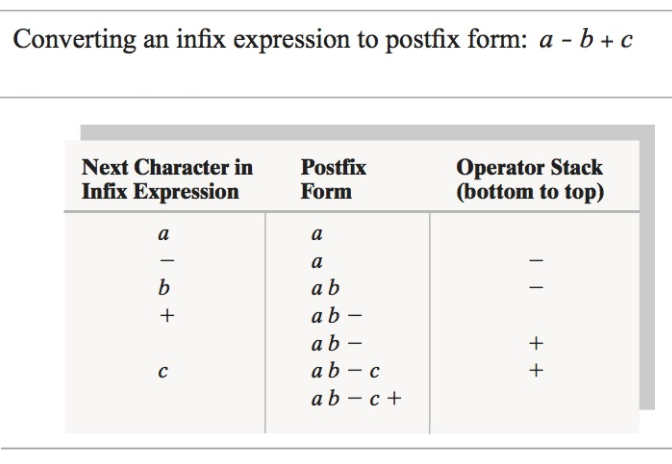# (Solved) : Use Postfix Reverse Polish Calculator Evaluate Infix Expressions Convert Infix Postfix For Q42687850 . . .

We can use the postfix (“Reverse Polish”) calculator to evaluateinfix expressions, once we convert an infix to its postfixform.

• To convert an infix expression to postfix form, we scan theinfix expression from left to right.
• When we encounter an operand, we place it at the end of the newexpression that we are creating
Operands in an infix expression remain in the same order in thecorresponding postfix expression.
• When we encounter an operator, we must save it until wedetermine where in the output expression it belongs.
For example, to convert the infix expression a +b, we append a to the initially emptyoutput expression, save +, and append b to theoutput expression.
We now need to retrieve the + and put it at theend of the output expression to get the postfix expressiona b +.
Retrieving the operator saved most recently is easy if we havesaved it in a stack.
In this example, we saved the operator until we processed itssecond operand.
• In general, we hold the operator in a stack at least until wecompare its precedence with that of the next operator.
For example, to convert the expression a + b * c,we append a to the output expression, push + ontoa stack, and then append b to the output.
What we do now depends on the relative precedences of the nextoperator, *, and the + at the topof the stack.
Since * has a greater precedence than+, b is not the addition’s secondoperand.
Thus, we push * onto the stack and appendc to the output expression.
• Having reached the end of the input expression, we now pop eachoperator from the stack and append it to the end of the outputexpression, getting the postfix expression a b c *+.
The figure shown here illustrates these steps.
The stack is shown horizontally; the leftmost element is at thebottom of the stack.
• What if two successive operators have the sameprecedence?
For example, consider the expression a – b +c.
When we encounter the +, the stack will containthe operator and the incomplete postfixexpression will be ab.
The subtraction operator belongs to the operands aand b, so we pop the stack and append to the end of the expression ab.
Since the stack is empty, we push the + onto thestack.
We then append c to the result, and finally we popthe stack and append the +.
The result is a b – c +.1. Implement this algorithm as a method with a String parameterrepresenting an arithmetic expression in infix notation, thatreturns the expression in postfix notation.
2. Your program (main method) should get an expression in infixnotation as user input, pass this expression to your method frompart (1), and then output the postfix expression (result of themethod call).

Converting an infix expression to postfix form: a – b + c Next Character in Infix Expression Postfix Form Operator Stack (bottom to top) TL olo + 0 ab – ab – + -C + -c+ Show transcribed image text Converting an infix expression to postfix form: a – b + c Next Character in Infix Expression Postfix Form Operator Stack (bottom to top) TL olo + 0 ab – ab – + -C + -c+

Answer to We can use the postfix (“Reverse Polish”) calculator to evaluate infix expressions, once we convert an infix to its post…

We are the best freelance writing portal. Looking for online writing, editing or proofreading jobs? We have plenty of writing assignments to handle.